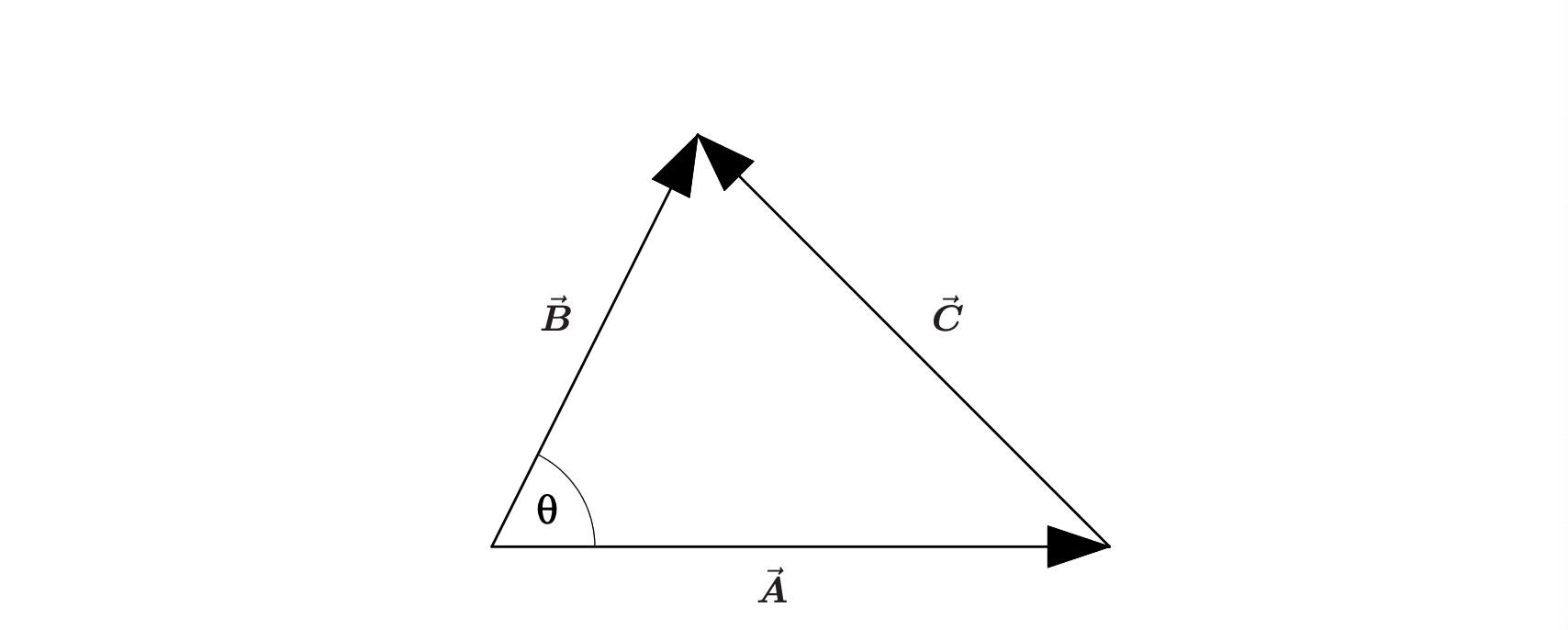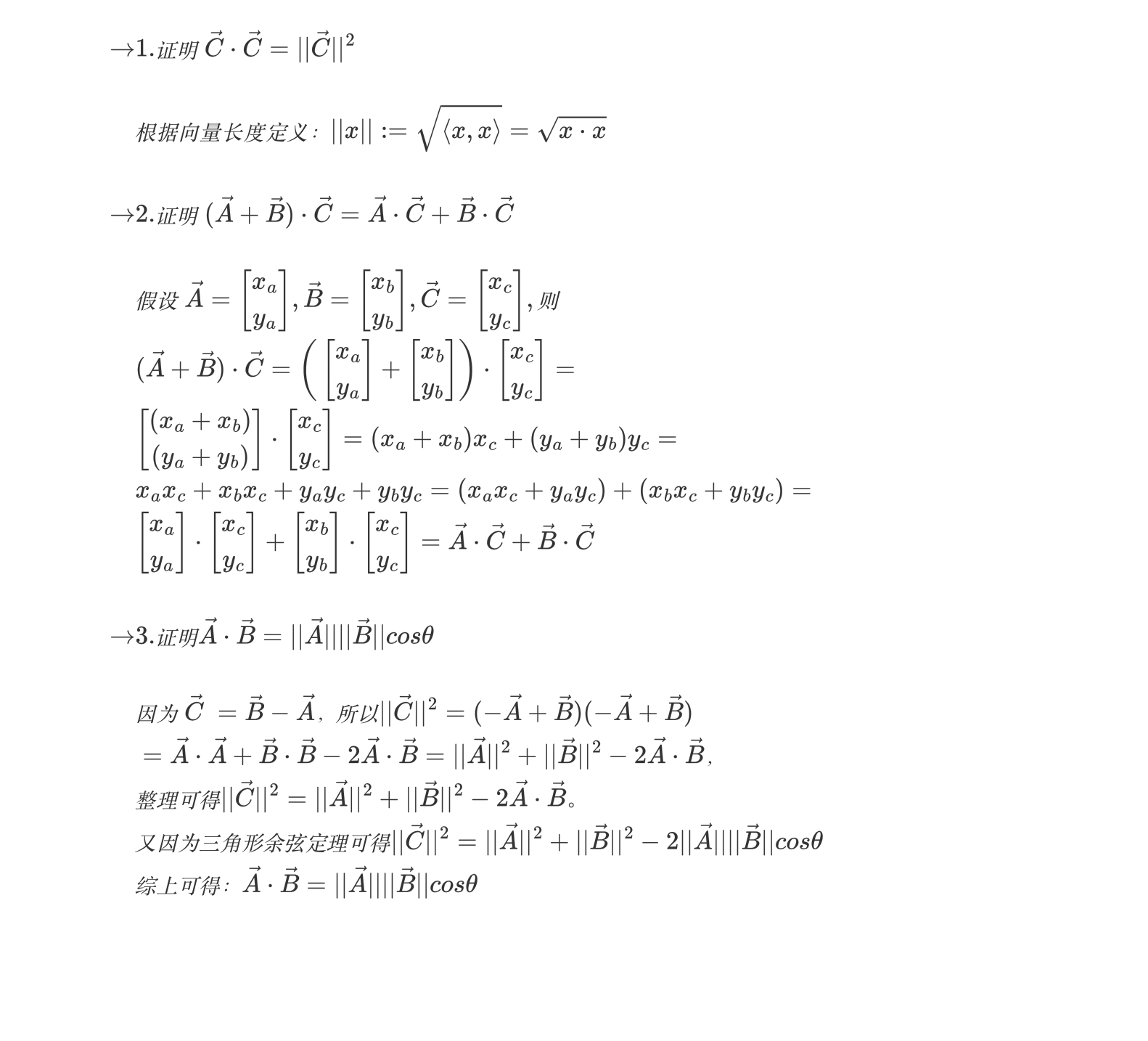• 参考： https://blog.csdn.net/dcrmg/article/details/52416832 ... 1 向量（点乘） ...a和b的点积（点乘）公式为： ...向量几何意义及用途 包括： 表征或计算两个向量之间的夹角 b向量在a向量...

参考：

https://blog.csdn.net/dcrmg/article/details/52416832

https://blog.csdn.net/qingzhuyuxian/article/details/84945319

1 向量内积（点乘）

公式

a和b的点积（点乘）公式为：向量内积的几何意义及用途

包括：

1. 表征或计算两个向量之间的夹角
2. b向量在a向量方向上的投影积，当a是单位向量时，内积意义是投影。
3. 当a是直线L的单位法向量时，计算b终点到L的距离

2 向量外积（叉乘）

2.1 公式：

对于向量a和向量b：a和b的外积公式为：几何意义及用途：

在三维几何中，向量a和向量b的外积结果是一个向量，有个更通俗易懂的叫法是法向量，该向量垂直于a和b向量构成的平面。

常用于以下情况：

1. 通过两个向量的外积，生成第三个垂直于a，b的法向量，从而构建X、Y、Z坐标系；
2. 当a是单位向量时，计算b终点到a所在直线的距离
3. 在二维空间中，aXb等于由向量a和向量b构成的平行四边形的面积。

展开全文向量
• 向量点积及其意义

千次阅读 2017-05-06 21:21:42
，定义它们的数量（又叫内点积）为以下实数： 更一般地，n维向量的内定义如下: 几何定义 设二维空间内有两个向量   和   ，它们的夹角为   ，则内定义为以下实数：...

定义

编辑

代数定义

设二维空间内有两个向量，定义它们的数量积（又叫内积、点积）为以下实数：更一般地，n维向量的内积定义如下:

几何定义

设二维空间内有两个向量，它们的夹角为，则内积定义为以下实数：该定义只对二维和三维空间有效。

应用

点乘的几何意义和用处就是计算两个向量之间的夹角，以及在某一方向上的投影。
在生产生活中，点积同样应用广泛。利用点积可判断一个多边形是否面向摄像机还是背向摄像机。向量的点积与它们夹角的余弦成正比，因此在聚光灯的效果计算中，可以根据点积来得到光照效果，如果点积越大，说明夹角越小，则物理离光照的轴线越近，光照越强。物理中，点积可以用来计算合力和功。若b为单位矢量，则点积即为a在方向b的投影，即给出了力在这个方向上的分解。功即是力和位移的点积。计算机图形学常用来进行方向性判断，如两矢量点积大于0，则它们的方向朝向相近；如果小于0，则方向相反。矢量内积是人工智能领域中的神经网络技术的数学基础之一，此方法还被用于动画渲染（Animation-Rendering）。
线性变换中点积的意义：
根据点积的代数公式：a·b=a1b1+a2b2+……+anbn，假设a为给定权重向量，b为特征向量，则a·b其实为一种线性组合，函数F（a·b）则可以构建一个基于a·b+c = 0 （c为偏移）的某一超平面的线性分类器，F是个简单函数，会将超过一定阈值的值对应到第一类，其它的值对应到第二类。

展开全文• 两个向量x,y∈Rnx,y \in{\Bbb R}^nx,y∈Rn的内​定义如下： ⟨x,y⟩:=x⋅y=∑i=1nxiyi \langle x,y \rangle := x \cdot y = \sum_{i=1}^n x_i y_i ⟨x,y⟩:=x⋅y=i=1∑n​xi​yi​ 即对两个向量执行对应位一一相乘...

两个向量 x , y ∈ R n x,y \in{\Bbb R}^n 的内积​定义如下：
⟨ x , y ⟩ : = x ⋅ y = ∑ i = 1 n x i y i \langle x,y \rangle := x \cdot y = \sum_{i=1}^n x_i y_i
即对两个向量执行对应位一一相乘再求和。如图，经过证明可以得到，即两个向量的内积（内乘）可以计算两个向量的夹角。

A ⃗ ⋅ B ⃗ = ∣ ∣ A ⃗ ∣ ∣ ∣ ∣ B ⃗ ∣ ∣ c o s θ \vec A \cdot \vec B = ||\vec A||||\vec B||cos\theta

证明过程如下：参考资料：

展开全文线性代数
• 向量 a⃗=(x1,y1)，b⃗=(x2,y2)\vec a=(x_1,y_1)，\vec b=(x_2,y_2)a=(x1​,y1​)，b=(x2​,y2​)，夹角为 θ\thetaθ，内为： a⃗⋅b⃗=∣a⃗∣∣b⃗∣cos⁡θ=x1x2+y1y2 \vec a \cdot \vec b=|\vec a||\vec b|...

一、点乘（内积）

有向量 a ⃗ = ( x 1 , y 1 ) ， b ⃗ = ( x 2 , y 2 ) \vec a=(x_1,y_1)，\vec b=(x_2,y_2) ，夹角为 θ \theta ，内积为：
a ⃗ ⋅ b ⃗ = ∣ a ⃗ ∣ ∣ b ⃗ ∣ cos ⁡ θ = x 1 x 2 + y 1 y 2 \vec a \cdot \vec b=|\vec a||\vec b|\cos\theta=x_1x_2 + y_1y_2

几何意义：
1. 夹角，由 a ⃗ ⋅ b ⃗ = ∣ a ⃗ ∣ ∣ b ⃗ ∣ cos ⁡ θ \vec a \cdot \vec b=|\vec a||\vec b|\cos\theta 知，当内积 > 0 >0 θ < 9 0 ∘ \theta<90^\circ ，内积 < 0 <0 θ > 9 0 ∘ \theta>90^\circ ，内积 = 0 =0 θ = 9 0 ∘ \theta=90^\circ 。同时也可以计算 θ \theta 的值： θ = a r c c o s a ⃗ ⋅ b ⃗ ∣ a ⃗ ∣ ∣ b ⃗ ∣ \theta=arccos\frac {\vec a \cdot \vec b}{|\vec a||\vec b|}
2. 投影 ∣ a ⃗ ∣ cos ⁡ θ = a ⃗ ⋅ b ⃗ ∣ b ⃗ ∣ |\vec a|\cos\theta=\frac {\vec a \cdot \vec b}{|\vec b|} 表示 a ⃗ \vec a b ⃗ \vec b 上的投影。
对偶性 a ⃗ ⋅ b ⃗ = ∣ a ⃗ ∣ ( ∣ b ⃗ ∣ cos ⁡ θ ) = ∣ b ⃗ ∣ ( ∣ a ⃗ ∣ cos ⁡ θ ) \vec a \cdot \vec b=|\vec a|(|\vec b|\cos\theta)=|\vec b|(|\vec a|\cos\theta)
∣ a ⃗ ∣ ( ∣ b ⃗ ∣ cos ⁡ θ ) |\vec a|(|\vec b|\cos\theta) 的理解是 a ⃗ \vec a 的长度与 b ⃗ \vec b a ⃗ \vec a 上的投影的乘积；
∣ b ⃗ ∣ ( ∣ a ⃗ ∣ cos ⁡ θ ) |\vec b|(|\vec a|\cos\theta) 的理解是 b ⃗ \vec b 的长度与 a ⃗ \vec a b ⃗ \vec b 上的投影的乘积；
而这两个是相等的。

二、叉乘（外积）上面的公式，就是求三阶行列式。

几何意义：
1. 上面如果不把 i ⃗ , j ⃗ , k ⃗ \vec i,\vec j,\vec k 的具体指带入公式，而是写成 a ⃗ × b ⃗ = m i ⃗ + n j ⃗ + l k ⃗ \vec a \times \vec b=m\vec i+n\vec j+l\vec k 的形式，向量 ( m , n , l ) (m,n,l) 就是一个同时垂直 a ⃗ \vec a b ⃗ \vec b 的向量，如下图：2. 对于二维向量， a ⃗ = ( x 1 , y 1 ) ， b ⃗ = ( x 2 , y 2 ) \vec a=(x_1,y_1)，\vec b=(x_2,y_2) ，按照上面的公式得：
a ⃗ × b ⃗ = ∣ x 1 y 1 x 2 y 2 ∣ = x 1 y 2 − x 2 y 1 \vec a \times \vec b=\begin{vmatrix} x_1 & y_1 \\ x_2 & y_2 \\ \end{vmatrix}=x_1y_2-x_2y_1 ，设这个数值为 m m
则， ∣ m ∣ = ∣ a × b ∣ = ∣ a ∣ ∣ b ∣ sin ⁡ θ |m|=|a×b|=|a| |b|\sin\theta θ \theta a ⃗ \vec a b ⃗ \vec b 的夹角）
且，|m| = a ⃗ \vec a b ⃗ \vec b 构成的平行四边形的面积 ，如下图：3. 判断向量的相对位置（顺逆时针）
a ⃗ \vec a b ⃗ \vec b 如图所示：如果让 a ⃗ \vec a 以最小角度转到 b ⃗ \vec b 的方向，是顺时针还是逆时针呢，从图中很容易看出，但怎么用数字判断呢？
仍然是 m = a ⃗ × b ⃗ = x 1 y 2 − x 2 y 1 m=\vec a \times \vec b=x_1y_2-x_2y_1
m > 0 m>0 a ⃗ \vec a 逆时针转到 b ⃗ \vec b 的角度 < 18 0 ∘ <180^\circ
m < 0 m<0 a ⃗ \vec a 逆时针转到 b ⃗ \vec b 的角度 > 18 0 ∘ >180^\circ
m = 0 m=0 a ⃗ \vec a b ⃗ \vec b 共线。

直观记忆如下图：m > 0 m>0 b ⃗ \vec b 在蓝色部分；
m < 0 m<0 b ⃗ \vec b 在红色部分；
m = 0 m=0 b ⃗ \vec b 在分界线上（与 a ⃗ \vec a 共线 ）。

三、扩展（坐标系引发的顺逆指针分不清事件）

我们平时默认的坐标系是这样的：但有时候的坐标系是这样的（比如数字图像中）：可以发现，同样的 a ⃗ = ( 2 , 1 ) \vec a=(2,1) 转到 b ⃗ = ( 1 , 2 ) \vec b=(1,2) ，在上面的坐标系中就是逆时针，而在下面的坐标系中就是顺时针，所以为了统一说明，定义了 “正旋转” ： x x 轴旋转到 y y 轴的方向。
所以，上面利用向量叉乘判断向量相对位置的性质描述应该为：
m > 0 m>0 a ⃗ \vec a 正旋转到 b ⃗ \vec b 的角度 < 18 0 ∘ <180^\circ
m < 0 m<0 a ⃗ \vec a 正旋转到 b ⃗ \vec b 的角度 > 18 0 ∘ >180^\circ
m = 0 m=0 a ⃗ \vec a b ⃗ \vec b 共线。
而那张直观记忆图只在我们平时默认的坐标系中才成立。

展开全文线性代数 向量点乘 向量内积 向量叉乘 向量外积
• 向量叉积的几何意义

万次阅读 多人点赞 2016-02-22 13:38:59
记得上大学时的第一节课是《空间解析几何》，和大多数的教材一样，开篇就是向量点积和叉积的定义。点积的定义很好理解 ，a·b（为了讨论方便，之后都假设b为单位向量）可以看成向量a在向量b方向上的投影长度。 ...
• 向量的内（点乘） ...a和b的点积公式为： 这里要求一维向量a和向量b的行列数相同。注意：点乘的结果是一个标量(数量而不是向量) 定义：两个向量a与b的内为a·b= |a||b|cos∠(a, b)，特别地，0·a=a·0= 0...内积
• 向量的基本几何意义

万次阅读 2019-03-06 11:24:25
自由向量： 大小和方向（物理：矢量） 向量的数学表示： 把空间中所有的向量的尾部都拉...向量加法的几何意义： 平行四边形法则、三角形法则 向量加法的物理意义： 船过河问题：船头的位移（马达动力）、流水...
• 1. 向量混合几何意义 2. 混合的应用示例1：已知四面体各顶点的坐标，求其体积 3.混合的应用示例2：四共面的充要条件是由其构成的三个向量的混合为零 ...
• 在3D游戏开发中，经常用到向量点积和叉积及其几何意义，为防止遗忘，在此记录一下。点积在数学中，点积(德语：Skalarprodukt、英语：Dot Product)又称数量或标量(德语：Skalarprodukt、英语：Scalar Product)...
• 点积的结果是一个标量，叉积的结果仍然是一个向量点积（dot product） 叉积（cross product） 向量长度： 向量方向：垂直于向量a和向量b构成的平面，方向满足右手法则！
• 向量是由n个实数组成的一个n行1列（n*1）或...向量的点乘,也叫向量的内、数量，对两个向量执行点乘运算，就是对这两个向量对应位一一相乘之后求和的操作，点乘的结果是一个标量。 点乘公式 对于向量a和向量b：向量点乘 向量积 法向量 内积
• 两个向量点积的几何意义

千次阅读 2013-04-09 20:35:43
一般而言点乘结果描述了两个向量的相似程度，点乘结果就越相近。
• 【线性代数的几何意义向量的基本几何意义 二、向量的基本几何意义 自由向量： 大小和方向（物理：矢量） 向量的数学表示： 把空间中所有的向量的尾部都拉到坐标原点，这样N维空间可以与N维向量空间建立一一...
• 向量的点乘,也叫向量的内、数量，对两个向量执行点乘运算，就是对这两个向量对应位一一相乘之后求和的操作，点乘的结果是一个标量。 点乘公式 对于向量a和向量b： &nbsp; &nbsp; &nbsp; &...向量 点乘
• 向量点乘（内向量点乘公式为： ...内（点乘）的几何意义： 表征或计算两个向量之间的夹角 b向量在a向量方向上的投影 判断两个向量是否同一方向或正交(即垂直)等方向关系，具体对应关系为：...向量点积 向量叉乘
• 给定向量a⃗,b⃗\vec a, \vec ba,b，夹角是θ\thetaθ（如下图），我们都知道，向量a⃗\vec aa和向量b⃗\vec bb的点积公式是 a⃗⋅b⃗=∥a⃗∥∥b⃗∥cos⁡θ\vec a \cdot \vec b=\|\vec a\|\|\vec b\|\cos{\theta}a...
• 向量点积 点积有两种好记好用的理解方法，他们都可以基于做功去理解： 假设b是个均匀分布的力场，a为收到其中作用进行的一个运动。 第一种基于几何： a点乘b等于一方（例b）投影到另一方（例a）的长度乘以另一方的...线性代数
• 向量的几何意义

千次阅读 2017-10-26 14:26:57
向量几何意义自由向量： 大小和方向（物理：矢量）向量的数学表示： 把空间中所有的向量的尾部都拉到坐标原点，这样N维空间可以与N维向量空间建立一一对应关系：N维空间中点(0，0，0…0)取作原点，那么每一...
• 二维向量叉乘：(x1,y1)×(x2,y2) = x1y2-x2y1 值为正，(x2,y2)在(x1,y1)逆时针方向 值为负，(x2,y2)在(x1,y1)顺时针方向 值为0，(x2,y2)和(x1,y1)共线 2.编程语言 # -*- coding: UTF-8 -*- from pylab import * x=...python 线性代数 机器学习
• 向量是由n个实数组成的一个n行1列（n*1）或一个1行n列（1*n）的有序数组；...向量的点乘,也叫向量的内、数量，对两个向量执行点乘运算，就是对这两个向量对应位一一相乘之后求和的操作，...向量 点乘 内积
• 对两个向量执行点乘运算，就是对这两个向量对应位一一相乘之后求和的操作，如下所示，对于向量a和向量b： a和b的点积公式为：这里要求一维向量a和向量b的行列数相同。注意：点乘的结果是一个标量(数量而不是向量)...
• 向量的内、外积及其几何含义

万次阅读 多人点赞 2019-03-18 15:37:51
一、向量的内（点乘） ...a和b的点积公式为： 这里要求一维向量a和向量b的行列数相同。注意：点乘的结果是一个标量(数量而不是向量) 定义：两个向量a与b的内为a·b= |a||b|cos∠(a, b)，特别地，0·a=a·0...向量 内积
• 向量（点乘）和外（叉乘）概念及几何意义 向量（点乘）和外（叉乘）概念及几何意义...